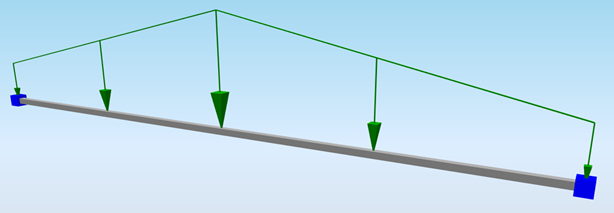# StructuralCurveAction¶

Line force on the beam

The Line force load models load distributed over a 1D member or on a slab edge. It may be action along the whole 1D member or only on its part. It can be constant or trapezoidal, acting in three main directions X, Y, Z (global or local coordinate system).Data type

Example / enum definition

Required

Description

Name

String

F3

yes

Human readable unique name of the force

Type

String

Standard

no

This property defines what the load is caused by, E.g. Standard, Wind, Snow, Self weight, Hoar Frost, Predefined, Plane Load, Water Pond, Water Pressure, Soil Pressure, Generated Water, Generated Soil

Force action

Enum

On beam

On edge

On subregion edge

On opening edge

On rib

yes

Specifies on which type of object the force acts

Distribution

Enum

Uniform

Trapez

yes

The load may be either constant along the 1D member or linearly variable (trapezoidal).

Direction

Enum

X

Y

Z

Vector

yes

Specifies the base direction of the load

X, Y, Z - action will be applied in one of these directions

Vector - size and direction calculated from vector

Value 1 [kN/m]

Double

-150

yes, if Direction = X,Y or Z

Specifies the first size of the load in, acts in one direction

Value1 is always closer to origin (see notes)

Value 2 [kN/m]

Double

-180

yes, if Direction = X,Y or Z

and Distribution = Trapez

Specifies the second size of the load in, acts in one direction

Value2 is always further from origin (see notes)

Vector 1(X;Y;Z) [kN/m]

String

(10;10;0)

yes, if Direction = Vector

Specifies the first size of the load in, direction by vector

Note: Vector1 and Vector2 needs to be in same direction

Vector 2(X;Y;Z) [kN/m]

String

(20;20;0)

yes, if Direction = Vector

and Distribution = Trapez

Specifies the second size of the load in, direction by vector

Note: Vector1 and Vector2 needs to be in same direction

Member

String

B11

yes, if Force action = On beam

The name of the StructuralCurveMember on which the load is applied

Member Rib

String

B11

yes, if Force action = On rib

The name of the StructuralCurveMemberRib on which the load is applied

2D Member

String

S1

yes, if Force action = On edge

The name of the StructuralSurfaceMember which the load is applied to. StructuralCurveAction can act either on a 2D member edge or on internal edge.

2D Member Region

String

R1

yes, if Force action = On subregion edge

The name of the StructuralSurfaceMemberRegion on which is the load applied.

2D Member Opening

String

O1

yes, if Force action = On opening edge

The name of the StructuralSurfaceMemberOpening on which is the load applied.

Edge

Integer

1

yes, if Force action = On edge, On subregion edge or On opening edge

The index starting with 1. The order is according to order of “edges” property at StructuralSurfaceMember, StructuralSurfaceMemberRegion or StructuralSurfaceMemberOpening on which the load is applied

Internal edge

String

ES2

yes, if Force action = On edge and Edge column has no value

The name of the StructuralCurveEdge on which the load is applied. StructuralCurveAction can act either on a 2D member edge or on internal edge.

String

LC1

yes

The name of the load case to which the force belongs

Coordinate system

Enum

Global

Local

yes

Defines the co-ordinate system of the member in which the load is applied

For ” Local”, coordinate system is defined by the member where is load applied

Location

Enum

Length

Projection

yes

Specifies whether the load is “put directly on an inclined 1D member” or whether the “projection on plan” is defined.

Coordinate definition

Enum

Absolute

Relative

yes

Selects the coordinate system that is used to define the length of the line load. Relative means without units. To define length of the load in meters input absolute

Origin

Enum

From start

From end

yes

Specifies the origin of the coordinate system used for the definition of the length of the force

Extent

Enum

Full

Span

yes

Defines if a load is extended just over a span instead of the whole 1D member, it is used if a 1D member consists of more than one span. This feature is not fully supported, only two spans are supported in the moment.

Start point [m]

Double

value in meters for Coordinate definition = Absolute

0,0

value in percentage for Coordinate definition = Relative

0,0

yes

Defines the position of the start point of the force in relative or absolute coordinates [m]

End point [m]

Double

value in meters for Coordinate definition = Absolute

5,25

value in percentage for Coordinate definition = Relative

1,0

yes

Defines the position of the end point of the force in relative or absolute coordinates [m]

Eccentricity ey [mm]

Double

-150

yes

Eccentricity of the force system line in Y direction of the local coordinate system

Eccentricity ez [mm]

Double

75

yes

Eccentricity of the force system line in Z direction of the local coordinate system

Parent ID

String

67b35d84-3d04-47aa-aa4a-dc1263982320

no

Is filled for objects created be dividing curved geometry to series of straight line objects.

Parent ID will ensure that curved edge is imported as straight parts to nonsupporting application, and back to original supporting application as curved geometry.

To ensure successful round trip of segmented objects and their related objects, Parent ID needs to be present in both directions.

Id

String

39f238a5-01d0-45cf-a2eb-958170fd4f39

no

Unique attribute designation